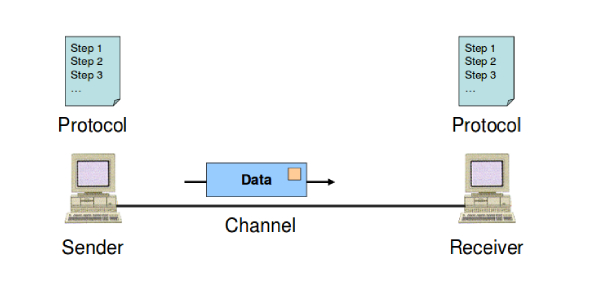# MCQ Quiz: Data Communication! Test

26 Questions | Attempts: 436
ShareSettingsThink you are an expert in digital communication? Can you pass this quiz? Data communication is the transmission of digital data between two or more computers and a computer network. It is a network that allows computers to exchange information. The physical connection between networked computing devices is established using either cable media or wireless media. Computer systems are connected to form a network. This quiz will explain all the variables of data communication.

• 1.
Sampling is process to convert _________ to ____________
• A.

Analog signal to digital signal

• B.

Continous time signal to discrete time signal

• C.

Signal to message

• D.

None of the above

• 2.
If even parity is being used then the value of parity bit should be ______ for data sequence 101010.
• A.

1

• B.

0

• C.

2

• D.

3

• 3.
Asynchronous transmission is ________ efficient as compared to synchronous transmission.
• A.

More

• B.

Equally

• C.

Less

• D.

None

• 4.
CRC code is ____________ technique.
• A.

Error detection

• B.

Error correction

• C.

Flow control

• D.

Encryption

• 5.
Consider data word 1110 is to transmitted using CRC code and if the pattern P= 1101 then how many redundant bits will be added to data word to make code word?
• A.

Three

• B.

Four

• C.

Two

• D.

One

• 6.
If the generator polynomial is X^4 + X^3 + 1 then the corresponding binary pattern will be:
• A.

11001

• B.

11010

• C.

10011

• D.

110

• 7.
The signal-to-noise ratio (in dB) for n-bit PCM can be expressed as:
• A.

6.02n + 1.76

• B.

20log 2 + 1.76

• C.

1.76n + 6.02

• D.

20nlog 2 + 1.76

• 8.
The ratio of data rate R, to transmission bandwidth is referred to as the:
• A.

Baud Rate

• B.

Bandwidth efficiency

• C.

Signal efficiency

• D.

Bit Rate Efficiency

• 9.
In asynchronous transmission, assume that data rate 10 kbps & the receiver clock is fast by 6%, then the receiver samples the incoming character at every:
• A.

100 micro seconds

• B.

47 micro seconds

• C.

94 micro seconds

• D.

50 micro seconds

• 10.
If R is the bit rate and r is related to the technique by which the signal is filtered to establish a bandwidth for transmission, then transmission bandwidth for ASK is equal to:
• A.

(1+ r)R

• B.

(1+ r)/R

• C.

(1+ R)r

• D.

(1+ R)/r

• 11.
The ________ between two words is the number of differences between corresponding bits.
• A.

HAMMING RULE

• B.

HAMMING DISTANCE HAMMING DISTANCE

• C.

HAMMING CODE

• D.

NONE OF THE ABOVE

• 12.
In block coding, we divide our message into blocks, is called
• A.

CODEWORDS

• B.

ERROR CODES

• C.

PACKET BLOCKS

• D.

DATAWORDS

• 13.
Find parity bit for 1001011.
• A.

1

• B.

2

• C.

3

• D.

0

• 14.
Which error detection method consists of just one redundant bit per data unit?
• A.

CRC

• B.

CheckSum

• C.

Simple Parity

• D.

Hamming Distance

• 15.
In ________, the amplitude of the carrier signal is varied to create signal elements. Both frequency and phase remain constant.
• A.

PSK

• B.

• C.

FSK

• D.

QAM

• 16.
_______ conversion is the process of changing one of the characteristics of an analog signal based on the information in the digital data.
• A.

Analog-to-analog

• B.

Digital-to-digital

• C.

Digital-to-Analog

• D.

None of these

• 17.
In ________, the phase of the carrier is varied to represent two or more different signal elements. Both peak amplitude and frequency remain constant.
• A.

PSK

• B.

• C.

FSK

• D.

QAM

• 18.
ASK, PSK, FSK, and QAM are examples of ________ conversion.
• A.

Analog-to-analog

• B.

Digital-to-digital

• C.

Analog-to-digital

• D.

Digital-to-analog

• 19.
If the value of checksum is 0, then the message is:
• A.

Accepted

• B.

Rejected

• C.

Sendback

• D.

None of these

• 20.
If the generator of cyclic code has at least two terms and rightmost bit is 1 then error cannot be divided by:
• A.

Syndrome

• B.

Error

• C.

Dataword

• D.

Generator

• 21.
In bit pattern, shifting to right means:
• A.

Adding extra 0s as rightmost bits

• B.

Deleting some rightmost bits

• C.

Adding extra 1s as rightmost bits

• D.

Deleting some leftmost bits

• 22.
The _______ of a polynomial is the highest power in the polynomial.
• A.

Range

• B.

Power

• C.

Degree

• D.

None

• 23.
In cyclic redundancy checking, the divisor is _______ the CRC.
• A.

The same size as

• B.

One bit less than one bit less than one bit less than one bit less than one bit less than

• C.

One bit more than

• D.

None

• 24.
In cyclic redundancy checking, what is the CRC?
• A.

The divisor The Divisor

• B.

The Dividend

• C.

The Reminder

• D.

The Quotient

• 25.
Which can detect two-bit errors?
• A.

Parity check

• B.

Parity & Cyclic redundancy check

• C.

Cyclic redundancy check

• D.

None of the mentioned

## Related TopicsBack to top
×

Wait!
Here's an interesting quiz for you.